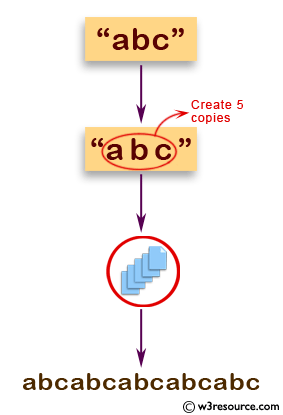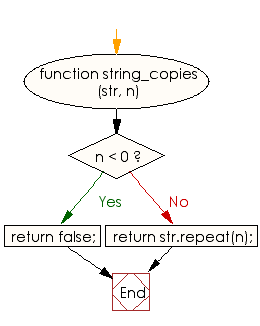# JavaScript: Create a new string of specified copies of a given string

## JavaScript Basic: Exercise-57 with Solution

Write a JavaScript program to create one string of specified copies (positive numbers) of a given string.

Pictorial Presentation:Sample Solution:

HTML Code:

``````<!DOCTYPE html>
<html>
<meta charset="utf-8">
<meta name="viewport" content="width=device-width">
<title>JavaScript program to create a new string of specified copies of a given string.</title>
<body>

</body>
</html>
```
```

JavaScript Code:

``````function string_copies (str, n)
{
if (n < 0)
return false;
else
return str.repeat(n);
}
console.log(string_copies("abc", 5));
console.log(string_copies("abc", 0));
console.log(string_copies("abc", -2));
```
```

Sample Output:

```abcabcabcabcabc

false
```

Flowchart:ES6 Version:

``````function string_copies (str, n)
{
if (n < 0)
return false;
else
return str.repeat(n);
}
console.log(string_copies("abc", 5));
console.log(string_copies("abc", 0));
console.log(string_copies("abc", -2));
``````

Live Demo:

See the Pen JavaScript - create a new string of specified copies (positive number) of a given string. - basic-ex-57 by w3resource (@w3resource) on CodePen.

What is the difficulty level of this exercise?

Test your Programming skills with w3resource's quiz.

﻿

## JavaScript: Tips of the Day

Calculates the greatest common divisor between two or more numbers/arrays

Example:

```const tips_gcd = (...arr) => {
const gcd = (x, y) => (!y ? x : tips_gcd(y, x % y));
return [...arr].reduce((p, q) => gcd(p, q));
};
console.log(tips_gcd(5, 25));
console.log(tips_gcd(...[8, 16, 32]));
```

Output:

```5
8
```

We are closing our Disqus commenting system for some maintenanace issues. You may write to us at reach[at]yahoo[dot]com or visit us at Facebook